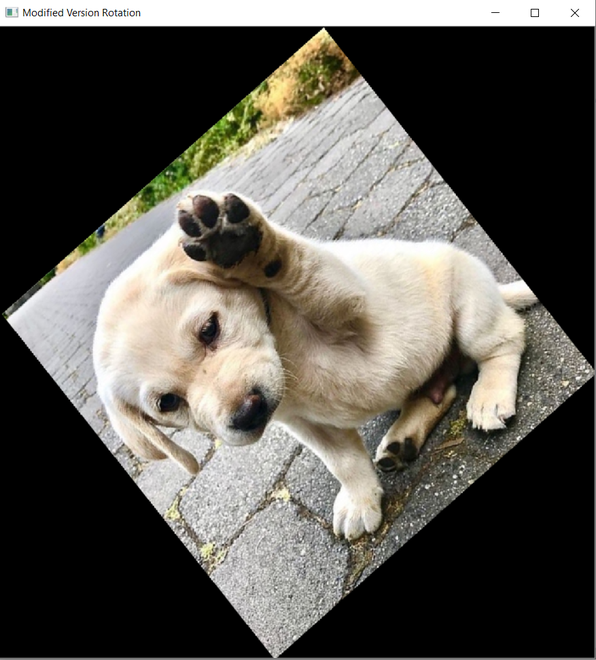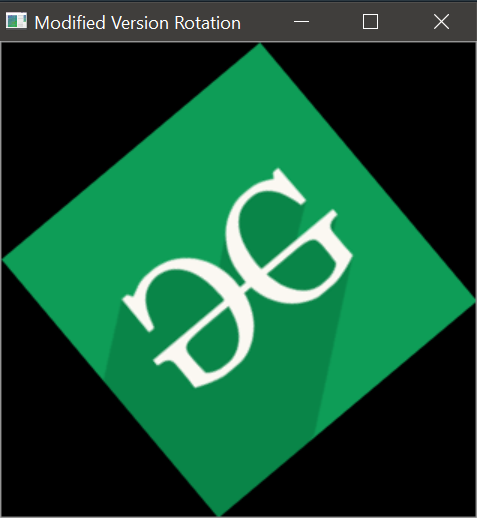# Rotate image without cutting off sides using Python – OpenCV

• Last Updated : 03 Nov, 2021

Rotating images with OpenCV is easy, but sometimes simple rotation tasks cropped/cut sides of an image, which leads to a half image.  Now, In this tutorial, We will explore a solution to safely rotate an image without cropping/cutting sides of an image so that the entire image will include in rotation, and also compare the conventional rotation method with the modified rotation version.

Step-by-step approach:

• In-order to rotate an image without cutting off sides, we will create an explicit function named ModifedWay() which will take the image itself and the angle to which the image is to be rotated as an argument.
• In the function, first, get the height and width of the image.
• Locate the center of the image.
• Then compute the 2D rotation matrix
• Extract the absolute sin and cos values from the rotation matrix.
• Get the new height and width of the image and update the values of the rotation matrix to ensure that there is no cropping.
• Finally, use the wrapAffine() method to perform the actual rotation of the image.

Below is the implementation of the approach:

## Python3

 `def` `ModifiedWay(rotateImage, angle):``   ` `    ``# Taking image height and width``    ``imgHeight, imgWidth ``=` `rotateImage.shape[``0``], rotateImage.shape[``1``]`` ` `    ``# Computing the centre x,y coordinates``    ``# of an image``    ``centreY, centreX ``=` `imgHeight``/``/``2``, imgWidth``/``/``2`` ` `    ``# Computing 2D rotation Matrix to rotate an image``    ``rotationMatrix ``=` `cv2.getRotationMatrix2D((centreY, centreX), angle, ``1.0``)`` ` `    ``# Now will take out sin and cos values from rotationMatrix``    ``# Also used numpy absolute function to make positive value``    ``cosofRotationMatrix ``=` `np.``abs``(rotationMatrix[``0``][``0``])``    ``sinofRotationMatrix ``=` `np.``abs``(rotationMatrix[``0``][``1``])`` ` `    ``# Now will compute new height & width of``    ``# an image so that we can use it in``    ``# warpAffine function to prevent cropping of image sides``    ``newImageHeight ``=` `int``((imgHeight ``*` `sinofRotationMatrix) ``+``                         ``(imgWidth ``*` `cosofRotationMatrix))``    ``newImageWidth ``=` `int``((imgHeight ``*` `cosofRotationMatrix) ``+``                        ``(imgWidth ``*` `sinofRotationMatrix))`` ` `    ``# After computing the new height & width of an image``    ``# we also need to update the values of rotation matrix``    ``rotationMatrix[``0``][``2``] ``+``=` `(newImageWidth``/``2``) ``-` `centreX``    ``rotationMatrix[``1``][``2``] ``+``=` `(newImageHeight``/``2``) ``-` `centreY`` ` `    ``# Now, we will perform actual image rotation``    ``rotatingimage ``=` `cv2.warpAffine(``        ``rotateImage, rotationMatrix, (newImageWidth, newImageHeight))`` ` `    ``return` `rotatingimage`

Below are some examples which depict how to rotate an image without cutting off sides using the above function:

Example 1:

Below is the implementation of the modified rotation version along with its comparison with normal rotation version:

## Python3

 `# Importing Required Libraries``import` `cv2``import` `numpy as np`` ` ` ` `# The below function is for conventionally way of rotating``# an Image without preventing cutting off sides``def` `SimpleWay(rotateImage, angle):``   ` `    ``# Taking image height and width``    ``imgHeight, imgWidth ``=` `rotateImage.shape[``0``], rotateImage.shape[``1``]`` ` `    ``# Computing the centre x,y coordinates``    ``# of an image``    ``centreY, centreX ``=` `imgHeight``/``/``2``, imgWidth``/``/``2`` ` `    ``# Computing 2D rotation Matrix to rotate an image``    ``rotationMatrix ``=` `cv2.getRotationMatrix2D((centreY, centreX), angle, ``1.0``)`` ` `    ``# Now, we will perform actual image rotation``    ``rotatingimage ``=` `cv2.warpAffine(``        ``rotateImage, rotationMatrix, (imgWidth, imgHeight))`` ` `    ``return` `rotatingimage`` ` ` ` `# The Below function is a modified version of the``# conventional way to rotate an image without``# cropping/cutting sides.``def` `ModifiedWay(rotateImage, angle):``   ` `    ``# Taking image height and width``    ``imgHeight, imgWidth ``=` `rotateImage.shape[``0``], rotateImage.shape[``1``]`` ` `    ``# Computing the centre x,y coordinates``    ``# of an image``    ``centreY, centreX ``=` `imgHeight``/``/``2``, imgWidth``/``/``2`` ` `    ``# Computing 2D rotation Matrix to rotate an image``    ``rotationMatrix ``=` `cv2.getRotationMatrix2D((centreY, centreX), angle, ``1.0``)`` ` `    ``# Now will take out sin and cos values from rotationMatrix``    ``# Also used numpy absolute function to make positive value``    ``cosofRotationMatrix ``=` `np.``abs``(rotationMatrix[``0``][``0``])``    ``sinofRotationMatrix ``=` `np.``abs``(rotationMatrix[``0``][``1``])`` ` `    ``# Now will compute new height & width of``    ``# an image so that we can use it in``    ``# warpAffine function to prevent cropping of image sides``    ``newImageHeight ``=` `int``((imgHeight ``*` `sinofRotationMatrix) ``+``                         ``(imgWidth ``*` `cosofRotationMatrix))``    ``newImageWidth ``=` `int``((imgHeight ``*` `cosofRotationMatrix) ``+``                        ``(imgWidth ``*` `sinofRotationMatrix))`` ` `    ``# After computing the new height & width of an image``    ``# we also need to update the values of rotation matrix``    ``rotationMatrix[``0``][``2``] ``+``=` `(newImageWidth``/``2``) ``-` `centreX``    ``rotationMatrix[``1``][``2``] ``+``=` `(newImageHeight``/``2``) ``-` `centreY`` ` `    ``# Now, we will perform actual image rotation``    ``rotatingimage ``=` `cv2.warpAffine(``        ``rotateImage, rotationMatrix, (newImageWidth, newImageHeight))`` ` `    ``return` `rotatingimage`` ` ` ` `# Driver Code`` ` `# Loading an Image from Disk``DogImage ``=` `cv2.imread(``"doggy.png"``, ``1``)`` ` ` ` `# Performing 40 degree rotation``NormalRotation ``=` `SimpleWay(DogImage, ``40``)``ModifiedVersionRotation ``=` `ModifiedWay(DogImage, ``40``)`` ` `# Display image on Screen``cv2.imshow(``"Original Image"``, DogImage)`` ` `# Display rotated image on Screen``cv2.imshow(``"Normal Rotation"``, NormalRotation)``cv2.imshow(``"Modified Version Rotation"``, ModifiedVersionRotation)`` ` ` ` `# To hold the GUI screen and control until it is detected``# the input for closing it, Once it is closed``# control will be released``cv2.waitKey(``0``)`` ` `# To destroy and remove all created GUI windows from``#screen and memory``cv2.destroyAllWindows()`

Output:Original ImageNormal Rotation with SimpleWay() functionRotation with ModifiedWay() function

Example 2:

Here is another example that depicts the modern rotation method:

## Python3

 `# Importing Required Libraries``import` `cv2``import` `numpy as np`` ` ` ` `# The Below function is a modified version of the``# conventional way to rotate an image without``# cropping/cutting sides.``def` `ModifiedWay(rotateImage, angle):``   ` `    ``# Taking image height and width``    ``imgHeight, imgWidth ``=` `rotateImage.shape[``0``], rotateImage.shape[``1``]`` ` `    ``# Computing the centre x,y coordinates``    ``# of an image``    ``centreY, centreX ``=` `imgHeight``/``/``2``, imgWidth``/``/``2`` ` `    ``# Computing 2D rotation Matrix to rotate an image``    ``rotationMatrix ``=` `cv2.getRotationMatrix2D((centreY, centreX), angle, ``1.0``)`` ` `    ``# Now will take out sin and cos values from rotationMatrix``    ``# Also used numpy absolute function to make positive value``    ``cosofRotationMatrix ``=` `np.``abs``(rotationMatrix[``0``][``0``])``    ``sinofRotationMatrix ``=` `np.``abs``(rotationMatrix[``0``][``1``])`` ` `    ``# Now will compute new height & width of``    ``# an image so that we can use it in``    ``# warpAffine function to prevent cropping of image sides``    ``newImageHeight ``=` `int``((imgHeight ``*` `sinofRotationMatrix) ``+``                         ``(imgWidth ``*` `cosofRotationMatrix))``    ``newImageWidth ``=` `int``((imgHeight ``*` `cosofRotationMatrix) ``+``                        ``(imgWidth ``*` `sinofRotationMatrix))`` ` `    ``# After computing the new height & width of an image``    ``# we also need to update the values of rotation matrix``    ``rotationMatrix[``0``][``2``] ``+``=` `(newImageWidth``/``2``) ``-` `centreX``    ``rotationMatrix[``1``][``2``] ``+``=` `(newImageHeight``/``2``) ``-` `centreY`` ` `    ``# Now, we will perform actual image rotation``    ``rotatingimage ``=` `cv2.warpAffine(``        ``rotateImage, rotationMatrix, (newImageWidth, newImageHeight))`` ` `    ``return` `rotatingimage`` ` ` ` `# Driver Code``# Loading an Image from Disk``Image ``=` `cv2.imread(``"gfg.png"``, ``1``)`` ` ` ` `# Performing 40 degree rotation``ModifiedVersionRotation ``=` `ModifiedWay(Image, ``40``)`` ` `# Display image on Screen``cv2.imshow(``"Original Image"``, Image)`` ` `# Display rotated image on Screen``cv2.imshow(``"Modified Version Rotation"``, ModifiedVersionRotation)`` ` ` ` `# To hold the GUI screen and control until it is detected``# the input for closing it, Once it is closed``# control will be released``cv2.waitKey(``0``)`` ` `# To destroy and remove all created GUI windows from``#screen and memory``cv2.destroyAllWindows()`

Output:My Personal Notes arrow_drop_up